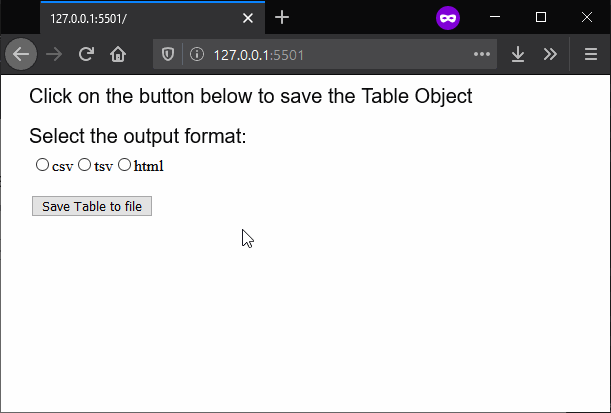# p5.js | saveTable() Function

• Last Updated : 16 Mar, 2020

The saveTable() function is used to save a p5.Table object to a file. The format of the file saved can be defined as a parameter to the function. By default, it saves a text file with comma-separated-values, however, it can be used to save it using-tab separated-values or generate an HTML table from it.

Syntax:

`saveTable( Table, filename, options )`

Parameters: This function accept three parameters as mentioned above and described below:

• Table: This is a p5.Table object that would be saved to the file.
• filename: It specifies the string that is used as the filename of the saved file.
• options: It is a string which denotes the format of the table to be saved. It can be either “csv” which saves the table using comma-separated-values, “tsv” which saves the table using tab-separated-values, or “html” which generates an HTML table. It is an optional parameter.

Below example illustrates the saveTable() function in p5.js:

Example:

 `function` `setup() { ` `  ``createCanvas(600, 300); ` `  ``textSize(20); ` `  `  `  ``text(``"Click on the button below to "` `        ``+ ``"save the Table Object"``, 20, 20); ` `   `  `  ``text(``"Select the output format:"``, 20, 60); ` `  `  `  ``// Create radio button for choosing ` `  ``// file format to save the table ` `  ``radio = createRadio(); ` `  ``radio.position(30, 80); ` `  ``radio.option(``'csv'``); ` `  ``radio.option(``'tsv'``); ` `  ``radio.option(``'html'``); ` `  `  `  ``// Create a button for saving the Table object ` `  ``saveBtn = createButton(``"Save Table to file"``); ` `  ``saveBtn.position(30, 120); ` `  ``saveBtn.mousePressed(saveFile); ` `  `  `  ``// Create the table for saving to file ` `  ``table = ``new` `p5.Table(); ` `  `  `  ``table.addColumn(``'Invention'``); ` `  ``table.addColumn(``'Inventors'``); ` `  `  `  ``let tableRow = table.addRow(); ` `  ``tableRow.setString(``'Invention'``, ``'Telescope'``); ` `  ``tableRow.setString(``'Inventors'``, ``'Galileo'``); ` `  `  `  ``tableRow = table.addRow(); ` `  ``tableRow.setString(``'Invention'``, ``'Steam Engine'``); ` `  ``tableRow.setString(``'Inventors'``, ``'James Watt'``); ` `  `  `  ``tableRow = table.addRow(); ` `  ``tableRow.setString(``'Invention'``, ``'Radio'``); ` `  ``tableRow.setString(``'Inventors'``, ``'Guglielmo Marconi'``); ` `} ` `  `  `function` `saveFile() { ` `  `  `  ``// Get the output format selected ` `  ``// from the radio buttons ` `  ``outputFormat = radio.value(); ` `  `  `  ``// Save the table to file with the given format ` `  ``saveTable(table, ``'tableOutput'``, outputFormat); ` `} `

Output:Online editor: https://editor.p5js.org/

My Personal Notes arrow_drop_up
Recommended Articles
Page :